# Edit Distance | (DP-33)

Problem Statement: Edit Distance

We are given two strings ‘S1’ and ‘S2’. We need to convert S1 to S2. The following three operations are allowed:

1. Deletion of a character.
2. Replacement of a character with another one.
3. Insertion of a character.

We have to return the minimum number of operations required to convert S1 to S2 as our answer.

```Example: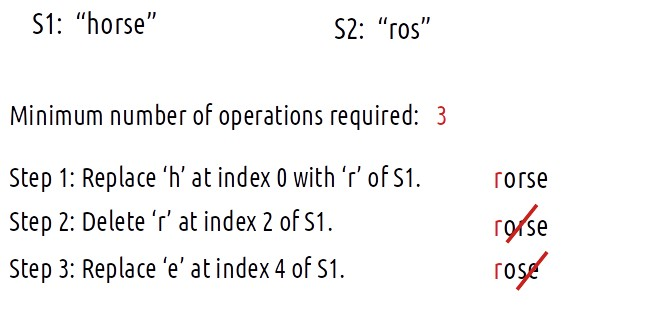```

Problem Link: Shortest Supersequence

Disclaimer: Don’t jump directly to the solution, try it out yourself first.

### Intuition:

For every index of string S1, we have three options to match that index with string S2, i.e replace the character, remove the character or insert some character at that index. Therefore, we can think in terms of string matching path as we have done already in previous questions.

As there is no uniformity in data, there is no other way to find out than to try out all possible ways. To do so we will need to use recursion.

Steps to form the recursive solution:

We will first form the recursive solution by the three points mentioned in the Dynamic Programming Introduction

##### Step 1: Express the problem in terms of indexes.

We are given two strings. We can represent them with the help of two indexes i and j. Initially, i=n-1 and j=m-1, where n and m are lengths of strings S1 and S2. Initially, we will call f(n-1,m-1), which means the minimum number of operations required to convert string S1[0…n-1] to string S2[0…m-1].

We can generalize this as follows: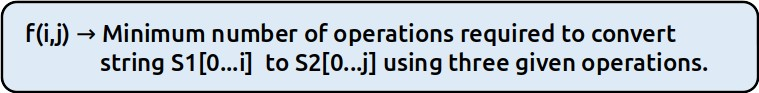##### Step 2: Try out all possible choices at a given index.

Now, i and j represent two characters from strings S1 and S2 respectively. There are only two options that make sense: either the characters represented by i and j match or they don’t.

(i) When the characters match

if(S1[i]==S2[j])

If this is true, now as the characters at i and j match, we would not want to do any operations to make them match, so we will just decrement both i and j by 1 and recursively find the answer for the remaining string portion. We return 0+f(i-1,j-1). The following figure makes it clear.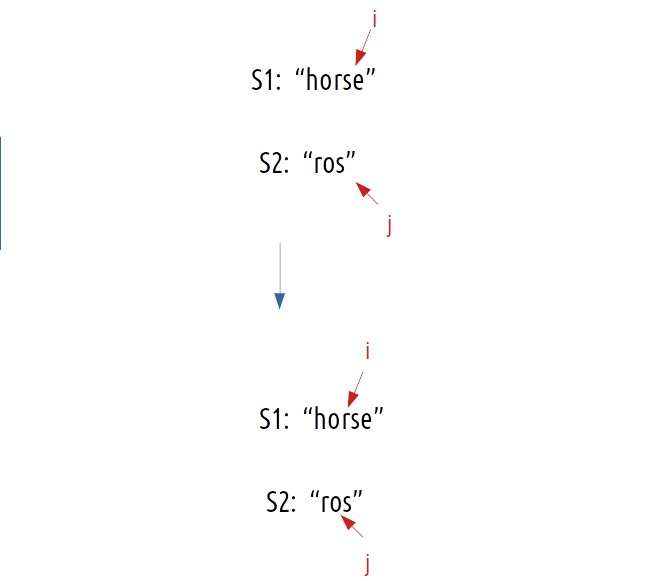(ii) When the characters don’t match

if(S1[i] != S2[j]) is true, then we have to do any of three operations to match the characters. We have three options, we will analyze each of them one by one.

Case 1: Inserting a character

Consider this example,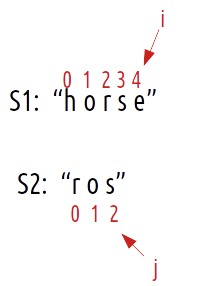Now if we have to match the strings by insertions, what would we do?:

• We would have placed an ‘s’ at index 5 of S1.
• Suppose i now point to s at index 5 of S1 and j points are already pointing to s at index j of S2.
• Now, we hit the condition, where characters do match. (as mentioned in case 1).
• Therefore, we will decrement i and j by 1. They will now point to index 4 and 1 respectively.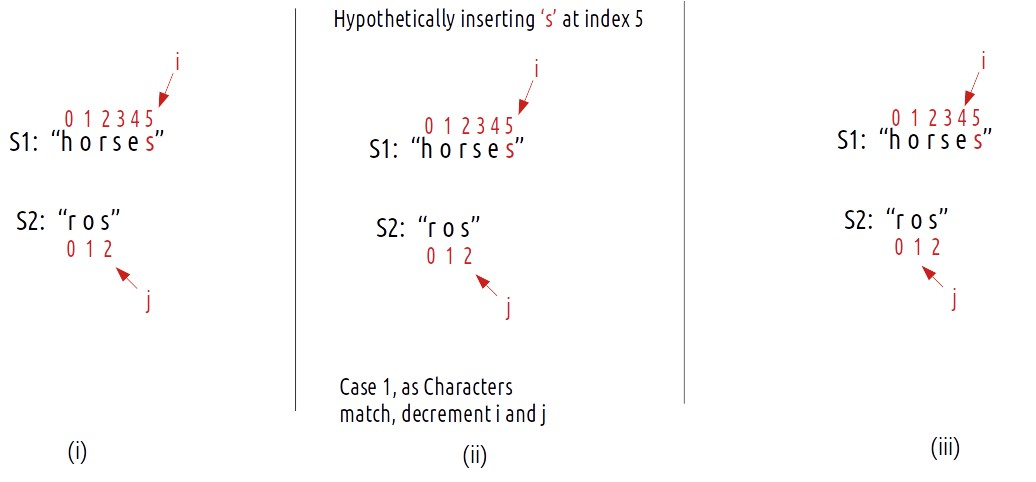Now, the number of operations we did were only 1 (inserting s at index 5) but do we need to really insert the ‘s’ at index 5 and modify the string? The answer is simply NO. As we see that inserting a character (here ‘s’ at index 5), we will eventually get to the third step. So we can just return 1+ f(i,j-1) as i remains there only after insertion and j decrements by 1. We can say that we have hypothetically inserted character s.

Case 2: Deleting a character

Consider the same example,We can simply delete the character at index 4 and check from the next index.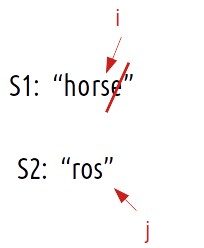Now, j remains at its original index and we decrement i by 1. We perform 1 operation, therefore we will recursively call 1+f(i-1,j).

Case 3: Replacing a character

Consider the same example,If we replace the character ‘e’ at index 4 of S1 with ‘s’, we have matched both the characters ourselves. We again hit the case of character matching, therefore we decrement both i and j by 1. As the number of operations performed is 1, we will return 1+f(i-1,j-1).

To summarise, these are the three choices we have in case characters don’t match:

• return 1+f(i-1,j) // Insertion of character.
• return 1+f(i,j-1) // Deletion of character.
• return 1+f(i-1,j-1) // Replacement of character.
##### Step 3: Return the minimum of all choices.

As we have to return the minimum number of operations, we will return the minimum of all operations.

Base Cases:

We are reducing i and j in our recursive relation, there can be two possibilities, either i becomes -1 or j becomes -1., i,e we exhaust either S1 or S2 respectively.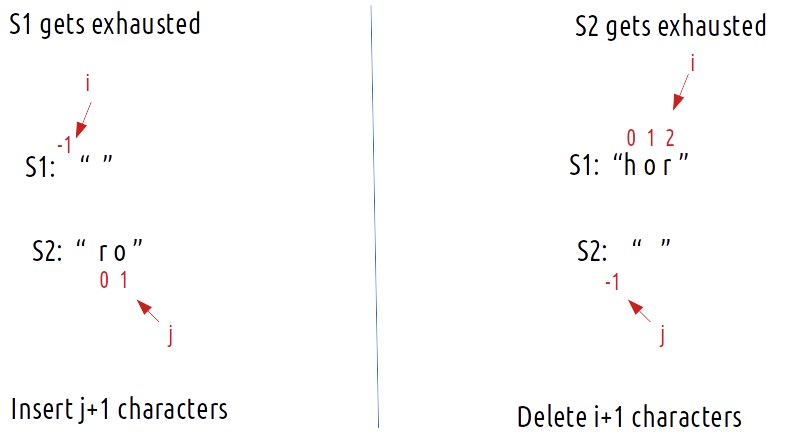The final pseudocode after steps 1, 2, and 3: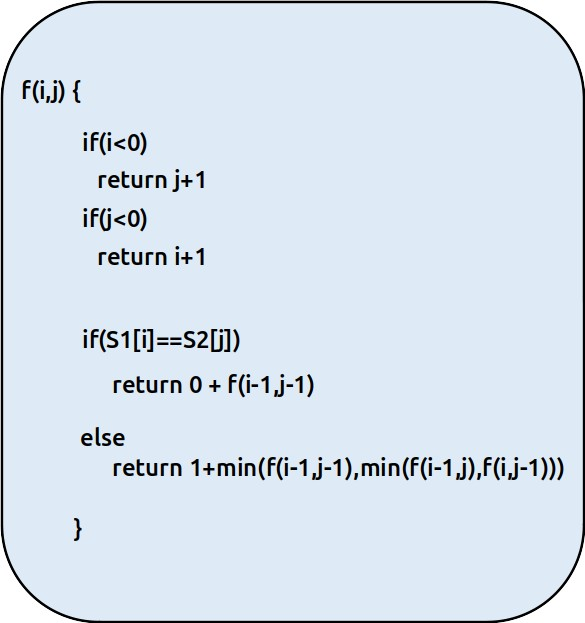Steps to memoize a recursive solution:

If we draw the recursion tree, we will see that there are overlapping subproblems. In order to convert a recursive solution the following steps will be taken:

1. Create a dp array of size [n][m]. The size of S1 and S2 are n and m respectively, so the variable i will always lie between ‘0’ and ‘n-1’ and the variable j between ‘0’ and ‘m-1’.
2. We initialize the dp array to -1.
3. Whenever we want to find the answer to particular parameters (say f(i,j)), we first check whether the answer is already calculated using the dp array(i.e dp[i][j]!= -1 ). If yes, simply return the value from the dp array.
4. If not, then we are finding the answer for the given value for the first time, we will use the recursive relation as usual but before returning from the function, we will set dp[i][j] to the solution we get.

Code:

## C++ Code

``````#include <bits/stdc++.h>
using namespace std;

int editDistanceUtil(string& S1, string& S2, int i, int j, vector<vector<int>>& dp){

if(i<0)
return j+1;
if(j<0)
return i+1;

if(dp[i][j]!=-1) return dp[i][j];

if(S1[i]==S2[j])
return dp[i][j] =  0+editDistanceUtil(S1,S2,i-1,j-1,dp);

// Minimum of three choices
else return dp[i][j] = 1+min(editDistanceUtil(S1,S2,i-1,j-1,dp),
min(editDistanceUtil(S1,S2,i-1,j,dp),editDistanceUtil(S1,S2,i,j-1,dp)));

}

int editDistance(string& S1, string& S2){

int n = S1.size();
int m = S2.size();

vector<vector<int>> dp(n,vector<int>(m,-1));
return editDistanceUtil(S1,S2,n-1,m-1,dp);

}

int main() {

string s1 = "horse";
string s2 = "ros";

cout << "The minimum number of operations required is: "<<editDistance(s1,s2);
}
``````

Output: The minimum number of operations required is: 3

Time Complexity: O(N*M)

Reason: There are N*M states therefore at max ‘N*M’ new problems will be solved.

Space Complexity: O(N*M) + O(N+M)

Reason: We are using a recursion stack space(O(N+M)) and a 2D array ( O(N*M)).

## Java Code

``````import java.util.*;

class TUF{
static int editDistanceUtil(String S1, String S2, int i, int j, int[][] dp){

if(i<0)
return j+1;
if(j<0)
return i+1;

if(dp[i][j]!=-1) return dp[i][j];

if(S1.charAt(i)==S2.charAt(j))
return dp[i][j] =  0+editDistanceUtil(S1,S2,i-1,j-1,dp);

// Minimum of three choices
else return dp[i][j] = 1+Math.min(editDistanceUtil(S1,S2,i-1,j-1,dp),
Math.min(editDistanceUtil(S1,S2,i-1,j,dp),editDistanceUtil(S1,S2,i,j-1,dp)));

}

static int editDistance(String S1, String S2){

int n = S1.length();
int m = S2.length();

int[][] dp=new int[n][m];
for(int row[]: dp)
Arrays.fill(row,-1);
return editDistanceUtil(S1,S2,n-1,m-1,dp);

}

public static void main(String args[]) {

String s1 = "horse";
String s2 = "ros";

System.out.println("The minimum number of operations required is: "+
editDistance(s1,s2));
}
}``````

Output: The minimum number of operations required is: 3

Time Complexity: O(N*M)

Reason: There are N*M states therefore at max ‘N*M’ new problems will be solved.

Space Complexity: O(N*M) + O(N+M)

Reason: We are using a recursion stack space(O(N+M)) and a 2D array ( O(N*M)).

Steps to convert Recursive Solution to Tabulation one.

In the recursive logic, we set the base case too if(i<0 ) and if(j<0) but we can’t set the dp array’s index to -1. Therefore a hack for this issue is to shift every index by 1 towards the right.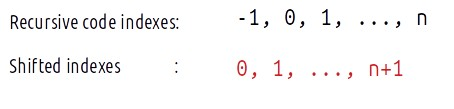• First we initialise the dp array of size [n+1][m+1] as zero.
• Next, we set the base condition (keep in mind 1-based indexing), we set the first column’s value as i and the first row as j( 1-based indexing).
• Similarly, we will implement the recursive code by keeping in mind the shifting of indexes, therefore S1[i] will be converted to S1[i-1]. Same for S2.
• At last, we will print dp[N][M] as our answer.

Code:

## C++ Code

``````#include <bits/stdc++.h>

using namespace std;

int editDistance(string& S1, string& S2){

int n = S1.size();
int m = S2.size();

vector<vector<int>> dp(n+1,vector<int>(m+1,0));

for(int i=0;i<=n;i++){
dp[i] = i;
}
for(int j=0;j<=m;j++){
dp[j] = j;
}

for(int i=1;i<n+1;i++){
for(int j=1;j<m+1;j++){
if(S1[i-1]==S2[j-1])
dp[i][j] = 0+dp[i-1][j-1];

else dp[i][j] = 1+min(dp[i-1][j-1],min(dp[i-1][j],dp[i][j-1]));
}
}

return dp[n][m];

}

int main() {

string s1 = "horse";
string s2 = "ros";

cout << "The minimum number of operations required is: "<<editDistance(s1,s2);
}
``````

Output: The minimum number of operations required is: 3

Time Complexity: O(N*M)

Reason: There are two nested loops

Space Complexity: O(N*M)

Reason: We are using an external array of size ‘N*M’. Stack Space is eliminated.

## Java Code

``````import java.util.*;

class TUF{

static int editDistance(String S1, String S2){

int n = S1.length();
int m = S2.length();

int[][] dp=new int[n+1][m+1];
for(int i=0;i<=n;i++){
dp[i] = i;
}
for(int j=0;j<=m;j++){
dp[j] = j;
}

for(int i=1;i<n+1;i++){
for(int j=1;j<m+1;j++){
if(S1.charAt(i-1)==S2.charAt(j-1))
dp[i][j] = 0+dp[i-1][j-1];

else dp[i][j] = 1+Math.min(dp[i-1][j-1],Math.min(dp[i-1][j],dp[i][j-1]));
}
}

return dp[n][m];
}

public static void main(String args[]) {

String s1 = "horse";
String s2 = "ros";

System.out.println("The minimum number of operations required is: "+
editDistance(s1,s2));
}
}``````

Output: The minimum number of operations required is: 3

Time Complexity: O(N*M)

Reason: There are two nested loops

Space Complexity: O(N*M)

Reason: We are using an external array of size ‘N*M’. Stack Space is eliminated.

Part 3: Space Optimization

If we closely look the relation,

dp[i][j] =  min(dp[i-1][j-1],dp[i-1][j],dp[i][j-1])

We see that to calculate a value of a cell of the dp array, we need only the previous row values (say prev). So, we don’t need to store an entire array. Hence we can space optimize it.

### Approach:

We will space optimize in the following way:

• We take two rows ‘prev’ and ‘cur’.
• We initialize it to the base condition. Now, at starting the prev row needs to be initialized with its column value. Moreover, the cur variable whenever declared should have its first cell as a row value. (See the code).
• Next, we implement the memoization logic. We replace dp[i-1] with prev and dp[i] by cur.
• After every inner loop execution, we set prev=cur, for the next iteration.
• At last, we return prev[m] as our answer.

Code:

## C++ Code

``````#include <bits/stdc++.h>

using namespace std;

int editDistance(string& S1, string& S2){

int n = S1.size();
int m = S2.size();

vector<int> prev(m+1,0);
vector<int> cur(m+1,0);

for(int j=0;j<=m;j++){
prev[j] = j;
}

for(int i=1;i<n+1;i++){
cur=i;
for(int j=1;j<m+1;j++){
if(S1[i-1]==S2[j-1])
cur[j] = 0+prev[j-1];

else cur[j] = 1+min(prev[j-1],min(prev[j],cur[j-1]));
}
prev = cur;
}

return prev[m];

}

int main() {

string s1 = "horse";
string s2 = "ros";

cout << "The minimum number of operations required is:  "<<editDistance(s1,s2);
}
``````

Output: The minimum number of operations required is: 3

Time Complexity: O(N*M)

Reason: There are two nested loops.

Space Complexity: O(M)

Reason: We are using an external array of size ‘M+1’ to store two rows.

## Java Code

``````import java.util.*;

class TUF{

static int editDistance(String S1, String S2){

int n = S1.length();
int m = S2.length();

int[] prev=new int[m+1];
int[] cur=new int[m+1];

for(int j=0;j<=m;j++){
prev[j] = j;
}

for(int i=1;i<n+1;i++){
cur=i;
for(int j=1;j<m+1;j++){
if(S1.charAt(i-1)==S2.charAt(j-1))
cur[j] = 0+prev[j-1];

else cur[j] = 1+Math.min(prev[j-1],Math.min(prev[j],cur[j-1]));
}
prev = (int[])(cur.clone());
}

return prev[m];

}

public static void main(String args[]) {

String s1 = "horse";
String s2 = "ros";

System.out.println("The minimum number of operations required is: "+
editDistance(s1,s2));
}
}``````

Output: The minimum number of operations required is: 3

Time Complexity: O(N*M)

Reason: There are two nested loops.

Space Complexity: O(M)

Reason: We are using an external array of size ‘M+1’ to store two rows.

Special thanks to Anshuman Sharma for contributing to this article on takeUforward. If you also wish to share your knowledge with the takeUforward fam, please check out this article